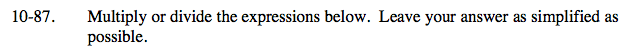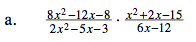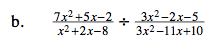### Home > AC > Chapter 10 > Lesson 10.2.4 > Problem10-87

10-87.For additional help with fractional expressions, see problem 10-65.$\frac{4(2x+1)(x-2)}{(x-3)(2x+1)}\cdot \frac{(x-3)(x+5)}{6(x-2)}$

This simplifies to the following:

$\frac{4(x+5)}{6}=\frac{2(x+5)}{3}$

$\frac{ 2(x + 5) }{3 }$$\frac{(7x-2)}{(x+4)}$